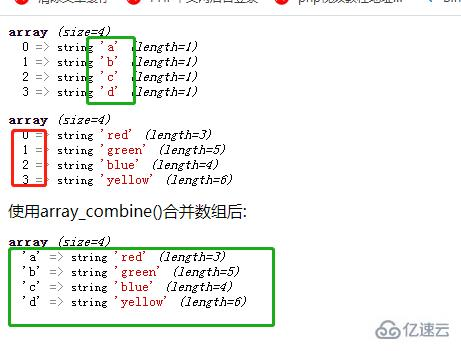# php怎么修改数组的下标php修改数组下标的两种方法

1、使用array_values() 函数

array_values() 函数可以将关联数组转化为索引数组，将字符串下标转为纯数字下标。

```<?php
\$arr=array("Peter"=>65,"Harry"=>80,"John"=>78,"Clark"=>90);
var_dump(\$arr);
var_dump(array_values(\$arr));
?>```2、使用array_combine()函数

array_combine()函数可以将一个数组的元素，作为另一个数组的键名（下标）。

```<?php
\$keys=array("a","b","c","d");
\$values=array("red","green","blue","yellow");
var_dump(\$keys);
var_dump(\$values);
echo "使用array_combine()合并数组后:";
var_dump(array_combine(\$keys,\$values));
?>``````<?php
\$keys=array("A","B","C","D");
\$values=array("a"=>"red","b"=>"green","c"=>"blue","d"=>"yellow");
var_dump(\$keys);
var_dump(\$values);
echo "使用array_combine()合并数组后:";
var_dump(array_combine(\$keys,\$values));
?>````array_combine(\$keys,\$values)`函数通过合并两个数组的方式创建了一个新数组，其中`\$keys`数组中的元素作为了新数组的键名，`\$values`数组的元素为作为了新数组的键值。

`\$keys`数组不可为多维数组，否则也会报错；但`\$values`数组可以为多维数组。